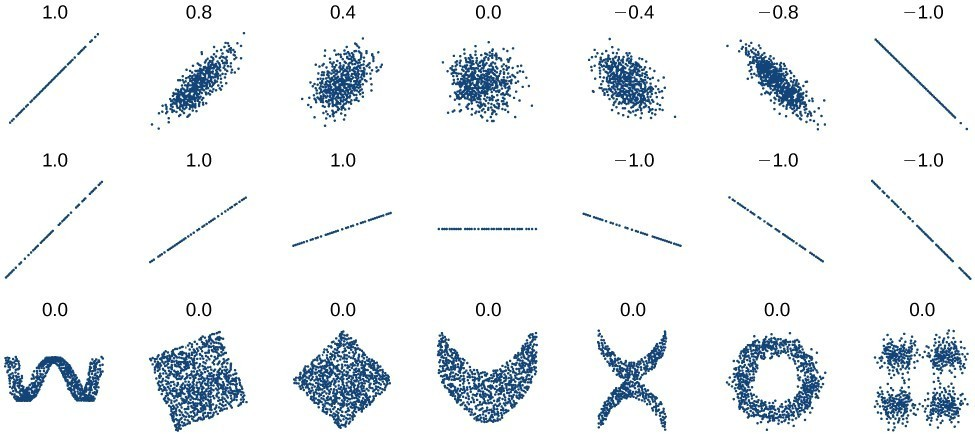## Distinguish between linear and nonlinear relations

As we saw in “Find the line of best fit” with the cricket-chirp model, some data exhibit strong linear trends, but other data, like the final exam scores plotted by age, are clearly nonlinear. Most calculators and computer software can also provide us with the correlation coefficient, which is a measure of how closely the line fits the data. Many graphing calculators require the user to turn a “diagnostic on” selection to find the correlation coefficient, which mathematicians label as r. The correlation coefficient provides an easy way to get an idea of how close to a line the data falls.

We should compute the correlation coefficient only for data that follows a linear pattern or to determine the degree to which a data set is linear. If the data exhibits a nonlinear pattern, the correlation coefficient for a linear regression is meaningless. To get a sense for the relationship between the value of r and the graph of the data, the image below shows some large data sets with their correlation coefficients. Remember, for all plots, the horizontal axis shows the input and the vertical axis shows the output.Figure 7. Plotted data and related correlation coefficients. (credit: “DenisBoigelot,” Wikimedia Commons)

### A General Note: Correlation Coefficient

The correlation coefficient is a value, r, between –1 and 1.

• r > 0 suggests a positive (increasing) relationship
• r < 0 suggests a negative (decreasing) relationship
• The closer the value is to 0, the more scattered the data.
• The closer the value is to 1 or –1, the less scattered the data is.

### Example 5: Finding a Correlation Coefficient

Calculate the correlation coefficient for cricket-chirp data in the table below.

 Chirps 44 35 20.4 33 31 35 18.5 37 26 Temperature 80.5 70.5 57 66 68 72 52 73.5 53

### Solution

Because the data appear to follow a linear pattern, we can use technology to calculate r. Enter the inputs and corresponding outputs and select the Linear Regression. The calculator will also provide you with the correlation coefficient, = 0.9509. This value is very close to 1, which suggests a strong increasing linear relationship.

Note: For some calculators, the Diagnostics must be turned “on” in order to get the correlation coefficient when linear regression is performed: [2nd]>>[alpha][x–1], then scroll to DIAGNOSTICSON.